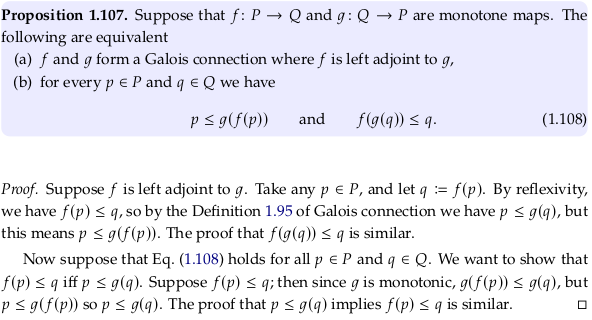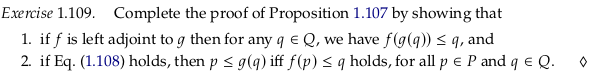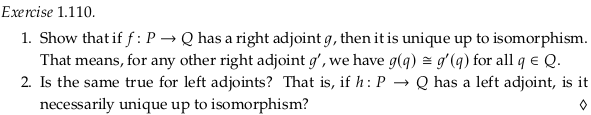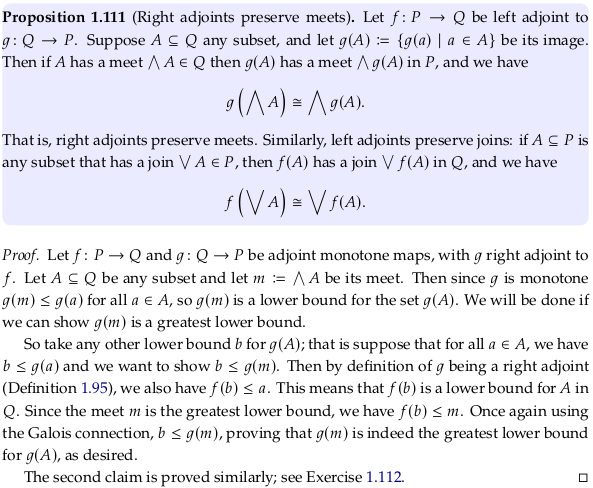# 1.4. Galois connections#

## 1.4.1. Definition and examples of Galois connections#

$\def\RR{{\bf R}} \def\ZZ{{\bf Z}}$

### Example 1.97.#

The first paragraph of this example should replace the dummy variable $$x$$ with $$y$$. Otherwise, it’s not clear in the next paragraph that $$x$$ is always a real number and $$y$$ is always an integer. The relation in this example needs to hold for any real number and integer; e.g. the pair (x=3.1, y=2) is always true, and (x=7.3, y=1) is always false. The author could have put this more precisely:

$\lceil x/3 \rceil \leq_\ZZ y \iff x \leq_\RR 3y$

Or as:

$\lceil r/3 \rceil \leq z \iff r \leq 3z$

See Floor and ceiling functions. By the definition of the ceiling function we have $$x \leq_\RR n \iff \lceil x \rceil \leq_\ZZ n$$. If we substitute $$x/3$$ for $$x$$, and $$y$$ for $$n$$, we get the result from the text.

### Exercise 1.98.#

We need a function from $$\RR \to \ZZ$$; an obvious function to try given the last example is $$\lfloor x/3 \rfloor$$.

See Floor and ceiling functions. By the definition of the floor function we have $$n \leq_\RR x \iff n \leq_\ZZ \lfloor x \rfloor$$. If we substitute $$x/3$$ for $$x$$, and $$y$$ for $$n$$, we get:

\begin{split} \begin{align} \\ y \leq_\RR x/3 \iff y & \leq_\ZZ \lfloor x/3 \rfloor \\ 3y \leq_\RR x \iff y & \leq_\ZZ \lfloor x/3 \rfloor \\ \end{align} \end{split}

As expected the right adjoint is $$\lfloor x/3 \rfloor$$.

### Exercise 1.99.#

All of these cases (for part 1.) are true, so $$f$$ is left adjoint to $$g$$:

\begin{split} \begin{align} \\ f(p=1) & = 1 \leq q=1 \iff p=1 \leq g(q=1) = 2 \\ f(p=2) & = 1 \leq q=1 \iff p=2 \leq g(q=1) = 2 \\ f(p=3) & = 3 \leq q=1 \iff p=3 \leq g(q=1) = 2 \\ f(p=1) & = 1 \leq q=2 \iff p=1 \leq g(q=2) = 2 \\ f(p=2) & = 1 \leq q=2 \iff p=2 \leq g(q=2) = 2 \\ f(p=3) & = 3 \leq q=2 \iff p=3 \leq g(q=2) = 2 \\ f(p=1) & = 1 \leq q=3 \iff p=1 \leq g(q=3) = 3 \\ f(p=2) & = 1 \leq q=3 \iff p=2 \leq g(q=3) = 3 \\ f(p=3) & = 3 \leq q=3 \iff p=3 \leq g(q=3) = 3 \\ \end{align} \end{split}

As we work through the cases for 2. we hit a failure:

\begin{split} \begin{align} \\ f(p=1) & = 1 \leq q=1 \iff p=1 \leq g(q=1) = 2 \\ f(p=2) & = 2 \leq q=1 \iff p=2 \leq g(q=1) = 2 \\ \end{align} \end{split}

### Remark 1.100.#Exercise 1.101.

We are interested in finding the function $$f$$:

$f(z) \leq r \iff z \leq \lceil r/3 \rceil$To fix the corner cases, one could define $$f(z)$$ to be the first real number greater than $$3(z-1)$$.

Looking forward, one could use Theorem 1.115 to define a left adjoint, although it would also be in an unsatisfying set-builder notation. Because $$g(r) = \lceil r/3 \rceil$$ preserves meets (trivial given it’s a total order), then by that theorem it should be a right adjoint.

The answer given in the Appendix seems wrong, with the logic failing at:

In the same way, $$L(1) \leq r$$ for all $$r > 0$$, so $$L(1) \leq 0$$.

## 1.4.2. Back to partitions#

between the preorder of $$S$$-partitions and …

Third paragraph, remove with:

if there exist $$s_1, s_2 \in S$$ such that

In the same paragraph, remove the unnecessary “?” in $$\sim_T^?$$.

### Exercise 1.103.#

The author asks for 6 examples in the question, then only bothers to do 4 in the Appendix. Doing so many is probably not a great use of time; this answer includes 5 only because the same drawings can be reused in the next question.### Exercise 1.105.#### Exercise 1.106.#

The parenthetical remark in 3. could be taken to mean you need to go back and fix your answer to 1. if you can’t answer 3., because you apparently misunderstood something. More likely it simply means you need to choose a partition in 1. that allows for both a coarser partition in 2. and a non-coarser partition in 3..## 1.4.3. Basic theory of Galois connections#

Second paragraph of proof, add to:

We want to show### Exercise 1.109.#Part 2., remove holds:

If Eq. (1.108) holds, then $$p \leq g(q) \iff f(p) \leq q$$

For 1. take any $$q \in Q$$ and let $$p := g(q)$$. With this definition we have $$p \leq g(q)$$, which by the definition of a Galois connection implies $$f(p) \leq q$$. Substituting the definition of $$p$$ in the last equation we get the desired result.

For 2. suppose $$p \leq g(q)$$. Since $$f$$ is monotonic we have $$f(p) \leq f(g(q))$$, and substituting $$f(g(q)) \leq q$$ we get the desired result $$f(p) \leq q$$.

### Exercise 1.110.#See the answer in the appendix; this answer would not be independent.

### Proposition 1.111#See Complete lattice § Morphisms of complete lattices for comments on the categories Sup (for supremum) and Inf (for infimum).

### Exercise 1.112.#

Let $$A \in P$$ be any subset and let $$j := \vee A$$ be its join. Then since $$f$$ is monotone $$f(a) \leq f(j)$$ for all $$a \in A$$, so $$f(j)$$ is an upper bound for the set $$f(A)$$. We must now show it is the least upper bound.

So take any other upper bound $$b$$ for $$f(A)$$; that is suppose that for all $$a \in A$$, we have $$f(a) \leq b$$ and we want to show $$f(j) \leq b$$. Then by definition of $$f$$ being a left adjoint we also have $$a \leq g(b)$$. However since $$j$$ is the least upper bound, we have $$j \leq g(b)$$. Again using the Galois connection, we have $$f(j) \leq b$$, proving that $$f(j)$$ really is the least upper bound for $$f(A)$$.

### Exercise 1.114.#

\begin{split} \begin{align} \\ f(p=1) & = 1 \leq q=1 \iff p=1 \leq g(q=1) = 1 \\ f(p=2) & = 2 \leq q=1 \iff p=2 \leq g(q=1) = 1 \\ f(p=3.9) & = 4 \leq q=1 \iff p=3.9 \leq g(q=1) = 1 \\ f(p=4) & = 4 \leq q=1 \iff p=4 \leq g(q=1) = 1 \\ f(p=1) & = 1 \leq q=2 \iff p=1 \leq g(q=2) = 2 \\ f(p=2) & = 2 \leq q=2 \iff p=2 \leq g(q=2) = 2 \\ f(p=3.9) & = 4 \leq q=2 \iff p=3.9 \leq g(q=2) = 2 \\ f(p=4) & = 4 \leq q=2 \iff p=4 \leq g(q=2) = 2 \\ f(p=1) & = 1 \leq q=4 \iff p=1 \leq g(q=4) = 4 \\ f(p=2) & = 2 \leq q=4 \iff p=2 \leq g(q=4) = 4 \\ f(p=3.9) & = 4 \leq q=4 \iff p=3.9 \leq g(q=4) = 4 \\ f(p=4) & = 4 \leq q=4 \iff p=4 \leq g(q=4) = 4 \\ \end{align} \end{split}

The second row in the solution for this question is incorrect.

### Theorem 1.115.#

In the third paragraph of the proof, replace 1.111 with 1.107:

By Proposition 1.107, it suffices

### Example 1.117.#

The names of the sets change from $$A$$/$$B$$ to $$X$$/$$Y$$ and then back again.

This section is confusing; the function $$f_{!}$$ is a left adjoint not to $$f_*$$ but $$f^*$$. Similarly $$f_{*}$$ is right adjoint to $$f^*$$, not $$f_!$$.

A similar example is given in category theory - A concrete intuition for Galois Connection - Math SE.

### Exercise 1.118.#

Selecting the function and subsets:\begin{split} \begin{align} f^*(B_1) & = \{a_1, a_2\} \\ f^*(B_2) & = \{a_3\} \\ f_!(A_1) & = \{b_1\} \\ f_!(A_2) & = \{b_1, b_2\} \\ f_*(A_1) & = \{b_1\} \\ f_*(A_2) & = \{b_2\} \end{align} \end{split}

## 1.4.4. Closure operators#

### Exercise 1.119.#

For 1., this is simply a restatement of the first part of $$p \leq g(f(p))$$ in Proposition 1.107. but with different symbols.

For 2., define $$q := f(p)$$. Applying $$f(g(q)) \leq q$$, we have $$f(g(f(p)) \leq f(p)$$. Applying the monotonicity property of $$g$$ we then have $$g(f(g(f(p)))) \leq g(f(p))$$, which is one half of what we are trying to prove.

Define $$p_1 := g(f(p))$$, and apply $$p_1 \leq g(f(p_1)$$ to get $$g(f(p)) \leq g(f(g(f(p))))$$, the second half of what we are trying to prove.

### Example 1.122.#

For the term “inclusion” used in this example, see Inclusion map.

It would be helpful to have an example to go with this example. See Upper set for the definition of an upper closure; let’s compute this closure on a simple preorder.### Which function is left and right?#

If you suspect or feel confident you have two functions that are adjoints, how do you determine which is left/right? In the examples in Section 1.4.1 above, the author establishes a convention that the left adjoint is a function from the top to bottom of a diagram and the right adjoint is a function from bottom to top. This doesn’t help if you don’t know which set to put on the top and bottom. Consider the following example:Recognize that the scales on your diagram don’t matter. You should be able to stretch/scale or shift to the left/right the scales on top and bottom.

Form what will be either a closure or interior operator by composing the two functions; in this case we’ll compose them as $$h_1=f⨟g$$ i.e. $$h_1=g∘f$$. On all pairs on top and bottom, check whether your composed function h is increasing (extensive per Closure operator) or decreasing. Similarly, form the opposite function $$h_2=f∘g$$ and apply the same tests.

If either function is not increasing or decreasing (both can be non-strictly), or what they do is not opposite, then you don’t have an adjoint pair. Choose whichever function is not an identity, and pattern match it against the definitions of a closure/interior operator in this section to determine which is left/right.

In the example above, $$h_1$$ is extensive and $$h_2$$ is the identity function. Therefore $$f$$ is the left adjoint, and $$g$$ is the right adjoint (this drawing isn’t actually too different than the one for Example 1.97 above).

## 1.4.5. Level shifting#

### Exercise 1.124.#Exercise 1.125.

For 1. define the preorder $$\leq$$:

$\begin{split} 1 \leq 3 \\ 2 \leq 3 \end{split}$

Then we have $$U(\leq) = \{(1,3), (2,3), (1,1), (2,2), (3,3)\}$$.

For 2. take the binary relations:

$\begin{split} Q = \{(1,3)\} \\ Q' = \{(3,1)\} \end{split}$

See Example 1.22 above; the authors are implying $$Cl$$ is a closure and therefore a left adjoint. In 3. we’ll only show that $$Cl$$ is monotone:

$Cl(Q) = Cl(\{1,3)\}) = Cl(\{(1,3), (1,1), (2,2), (3,3)\})$

The preorder $$Cl(Q)$$:

$1 \leq 3$

Clearly $$Cl(Q) \sqsubseteq \leq$$ (see $$\leq$$ defined in 1. above). For 4. we have:

$Cl(Q') = Cl(\{3,1)\}) = Cl(\{(3,1), (1,1), (2,2), (3,3)\})$

The preorder $$Cl(Q')$$:

$3 \leq 1$

Clearly $$Cl(Q) \not\sqsubseteq \leq$$.